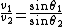# Refraction of waves

Also found in: Dictionary, Thesaurus, Medical.

## Refraction of waves

The change of direction of propagation of any wave phenomenon which occurs when the wave velocity changes. The term is most frequently applied to visible light, but it also applies to all other electromagnetic waves, as well as to sound and water waves.

The physical basis for refraction can be readily understood with the aid of the illustration. Consider a succession of equally spaced wavefronts approaching a boundary surface obliquely. The direction of propagation is in ordinary cases perpendicular to the wavefronts. In the case shown, the velocity of propagation is less in medium 2 than in medium 1, so that the waves are slowed down as they enter the second medium. Thus, the direction of travel is bent toward the perpendicular to the boundary surface (that is, Θ2 < Θ1). If the waves enter a medium in which the velocity of propagation is faster than in their original medium, they are refracted away from the normal.

#### Snell's law

The simple mathematical relation governing refraction is known as Snell's law. If waves traveling through a medium at speed v1 are incident on a boundary surface at angle Θ1 (with respect to the normal), and after refraction enter the second medium at angle Θ2 (with the normal) while traveling at speed v2, then

(1)Eq. (1) holds. The index of refraction n of a medium is defined as the ratio of the speed of waves in vacuum c to their speed in the medium. Thus c = n1v1 = n2v2, and therefore
(2)Eq. (2) holds. The refracted ray, the normal to the surface, and the incident ray always lie in the same place.

The relative index of refraction of medium 2 with respect to that of medium 1 may be defined as n = . Snell's law then becomes Eq. (3).

(3)For sound and other elastic waves which require a medium in which to propagate, only this last form has meaning. Equation (3) is frequently used for light when one medium is air, whose index of refraction is very nearly unity.

When the wave travels from a region of low velocity (high index) to one of high velocity (low index), refraction occurs only if (n1/n2) sin Θ1 &lsim; 1. If Θ1 is too large for this relation to hold, then sin Θ2 > 1, which is meaningless. In this case the waves are totally reflected from the surface back into the first medium. The largest value that Θ1 can have without total internal reflection taking place is known as the critical angle Θc. Thus sin Θc = .

#### Atmospheric refraction

The index of refraction of the Earth's atmosphere increases continuously from 1.000000 at the edge of space to 1.000293 (yellow light) at 0°C and 760 mmHg (101.3 kilopascals) pressure. Thus celestial bodies as seen in the sky are actually nearer to the horizon than they appear to be. The effect decreases from a maximum of about 35 minutes of arc for an object on the horizon to zero at the zenith, where the light enters the atmosphere at perpendicular incidence.

Other manifestations of atmospheric refraction are the mirages and “looming” of distant objects which occur over oceans or deserts, where the vertical density gradient of the air is quite uniform over a large area.

#### Sound waves

The velocity of sound in a gas is proportional to the square root of the absolute temperature. Because of the vertical temperature gradients in the atmosphere, refraction of sound can be quite pronounced. As in mirage formation, to allow large-scale refraction the temperature at a given height must be uniform over a rather large horizontal area. See Atmospheric acoustics, Sound

#### Seismic waves

The velocity of elastic waves in a solid depends upon the modulus of elasticity and upon the density of the material. Waves propagating through solid earth are refracted by changes of material or changes of density. Worldwide observations of earthquake waves enable scientists to draw conclusions on the distribution of density within the Earth.

#### Water waves

As the waves enter shallower water they travel more slowly. As a train of waves approaches a coastline obliquely, its direction of travel becomes more nearly perpendicular to the shore because of refraction. See Wave motion

McGraw-Hill Concise Encyclopedia of Physics. © 2002 by The McGraw-Hill Companies, Inc.
Site: Follow: Share:
Open / Close